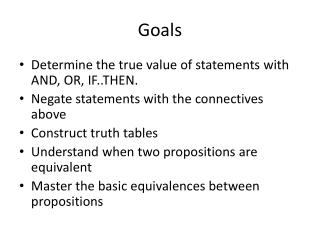Download PresentationGoals

# Goals - PowerPoint PPT Presentation

Goals. Determine the true value of statements with AND, OR, IF..THEN. Negate statements with the connectives above Construct truth tables Understand when two propositions are equivalent Master the basic equivalences between propositions. 2.1 Logical Form and Logical Equivalence.I am the owner, or an agent authorized to act on behalf of the owner, of the copyrighted work described.
Download Presentation## Goals

Download Policy: Content on the Website is provided to you AS IS for your information and personal use and may not be sold / licensed / shared on other websites without getting consent from its author.While downloading, if for some reason you are not able to download a presentation, the publisher may have deleted the file from their server.

- - - - - - - - - - - - - - - - - - - - - - - - - - E N D - - - - - - - - - - - - - - - - - - - - - - - - - -
Presentation Transcript
1. Goals • Determine the true value of statements with AND, OR, IF..THEN. • Negate statements with the connectives above • Construct truth tables • Understand when two propositions are equivalent • Master the basic equivalences between propositions

2. 2.1 Logical Form and Logical Equivalence The central concept of deductive logic is the concept of argument form. An argument is a sequence of statements aimed at demonstrating the truth of an assertion. The assertion at the end of the sequence is called the conclusion, and the preceding statements are called premises.

3. In logic what matters is the form of the argument, not its content • Charles will go overseas or finish school • 3 is greater than 2 or 3 is less than 5 Both statements have the form

4. A proposition (statement) is a sentence that is either true (T) or false (F) but not both • Today is Sunday • It is false since today is not Sunday • 2+2=4 • It is true Not statements • He is tall • He does not refer to any particular person • x>2

5. Compound Statements Statements are denoted by letters such as p, q, r, s t: Today is Sunday q: 2+2=4 Four symbols are used to build more complicated logical expressions out of simpler ones. ~ denotes not denotes and denotes or denotes implication (conditional)

6. Given a statement p • “~p” is read “not p” or “It is not the case that p” and is called the negation of p. In some computer languages the symbol  is used in place of ~. • Given statements p, q • “pq” is read “p and q” and is called the conjunction of p and q. • “pq” is read “p or q” and is called the disjunction of p and q. • "p q" is read " if p then q" and is called implication

7. COMMENTS • ~(pq) represents the negation of the conjunction of p and q. • an expression such as pqr is considered ambiguous.This expression must be written as either (pq) r orp (qr) to have meaning. EXERCISE: Write symbolically each of the statements below. Label propositions. – Today is hot but it is not humid – Chris will play chess or he will read a book – Jessica does not like shopping – If it is cloudy, it will rain – The car is neither expensive nor powerful.

8. TRUTH TABLES Tree to choose combination of truth values. False when both are false True when both are true

9. Exercise Construct the truth table for the statement form (pq)  ~(pq). Note: When or is used in its exclusive sense, the statement “p or q” means “p or q but not both” or “p or q and not both p and q,” which translates into symbols as (pq)  ~(pq). This is sometimes abbreviated

10. Logical Equivalent Propositions Two statements, P, Q, are equivalent if they have exactly the same truth value for any choice of truth value for the component statements. It is denoted P≡Q

11. Double Negative Property: ∼(∼p)≡ p p: Firrco is not blind ~p: ~(~p):

12. De Morgan’s laws Symbolically we can represent the two logic equivalences as: and Use truth tables to show they are equivalent.

13. Write negations for each of the following statements: a. John is 6 feet tall and he weighs at least 200 pounds. b. The bus was late or Tom’s watch was slow. • Solution: a. John is not 6 feet tall or he weighs less than 200 pounds. b. The bus was not late and Tom’s watch was not slow. Or neither was the bus late nor was Tom’s watch slow.

14. Logically Non-Equivalent Statements Show that the statement forms ~(pq) and ~p ~q are not logically equivalent Solution: a. This method uses a truth table annotated with a sentence of explanation.

15. Knowledge of logically equivalent statements is very useful for constructing arguments. It often happens that it is difficult to see how a conclusion follows from one form of a statement, whereas it is easy to see how it follows from a logically equivalent form of the statement.

16. Summary of Logical Equivalences • A number of logical equivalences are summarized in Theorem 2.1.1 for future reference.

17. Use Theorem 2.1.1 to verify the logical equivalence

18. Tautology and Contradiction A tautology is a proposition that is always true. To be or not to be A contradiction is a proposition that is always false. To be and not to be